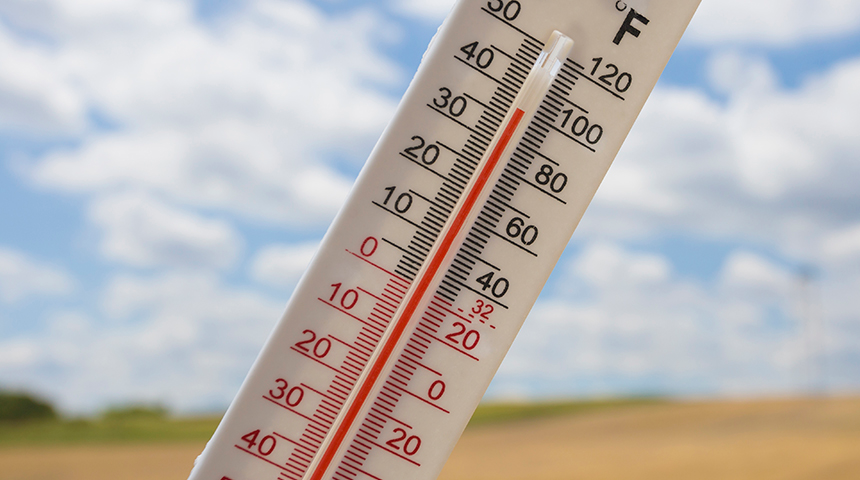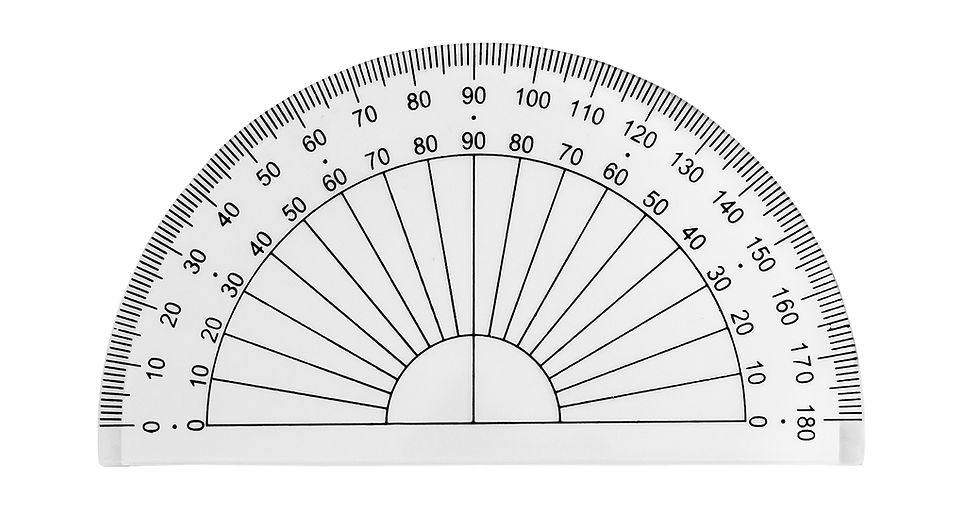top of page

#1 App for all working in the trades! Training, certifications & tools.

Search

# HVAC Trade Math: Unit Systems & Measurement

## In this modulus, we will introduce the two-unit systems in science and mathematics. Skip to quiz!

### Introduction to Units

Say we measure the length of a pipe and write the length as 10. We don’t have the unit with the number. Without it, we don’t know the pipe’s length. With units, we can know if the pipe is 10 inches long or 10 meters long. Here, inches and meters are units.

Scientists started using units in the late 17th century. Since then, two-unit systems have been created to define measurements.

The two systems of measurements are:

1. Imperial Unit System

2. Metric Unit System

The rest of the module, we will discuss the different units in the systems and how to convert between the two-unit systems.The two-unit systems let us measure things in many different ways. Each unit system has its units for length, area, volume and weight. For example, we can measure the length in inches, centimeters or meters, as we will see later on in the unit.

Before looking at different units for length, area, volume, weight and mass, let us recall these measurements.

Length is the distance between two points. In the picture shown, the length between one side of the square to another is 8 inches.

Area is the amount of space a shape takes up on a flat surface. 2D shapes have two dimensions: length and width. This is why the area is calculated using squared units.

.

Volume is the space a three-dimensional shape takes up. 3D shapes have three dimensions: length, width and thickness. Volume is calculated in cubic units.

Mass is the amount of substance something has. For example, when we fill an empty bottle with water, we make the bottle heavier. Here, water is the substance. The more substance something has, the heavier it is.

In this unit, we learned about the importance of units. We also introduced the two-unit systems: imperial and metric unit systems

## Previously we have introduced the unit of measurements: imperial and metric. In this unit, we will look at different units in each system for various measurements. We will also look at how to convert between the unit systems. Skip to quiz!

### Imperial System

The imperial measurement is only used in the United States. Let’s look at the imperials units of length, area, volume and mass.

The imperial units for measuring length are as follows:

• Inch (in)

• Foot (ft)

• Yard (yd)

• Mile (mi)

Let us take a look at how these units are related to one another.

The imperial units for the area are as follows:

• Square Inch ( in²)

• Square Foot (ft²)

• Acre (ac)

• Square Mile (mi²)

Similar to length, units for the area are also related. Here, we are shown that 1 in² is equal to the face of a dice. We are also shown that 1 ft² is equal to a garden square. We can also say that 1 ft² is equal to having 144 in².

Here, we are shown that 1 acre of land is equivalent to little more than half of a soccer field. We are also shown that 1 mil² is about the size of the central park. It can also be written as 1 mil² is equal to 640 acres.

The imperial units for volume are as follows:

• Fluid Ounce (fl oz.)

• Cup

• Pint

• Quart (qt)

• Gallon (gal)

The following are the imperial units of weight.

• Ounce (oz)

• Pound (lb)

• Ton

### Metric System

Almost all countries use the metric system. Like the imperial system, the metric system also has length, area, volume, and mass.

The metric units for measuring length are as follows:

• Millimeter (mm)

• Centimeter (cm)

• Meter (m)

• Kilometer (km)Let us take a look at how these units are related to one another.

The metric units for the area are as follows:

• Square Millimeter (mm²)

• Square Centimeter (cm²)

• Hecta (ha)

• Square Meter (m²)

• Square Kilometre (km²)

The metric units for volume are as follows:

• Cubic Millimeter (mm³)

• Cubic Centimeter (cm³)

• Millilitre (ml)

• Litre (L)

• Cubic Meter (m³)

The following are the imperial units of weight.

• Gram (g)

• Kilogram (kg)

### Unit Conversion

When converting between unit systems, the easiest way is by using google.Here, we are shown what to write in the search bar when converting from metric to imperial units. We write the metric unit we want to convert first. Then, we write the imperial unit we want to convert to. Here, we are converting 100 in to mm.

When we hit search, two boxes will appear. Here, we are shown that the box on the left is the metric unit we are converting. The box on the right is the converted imperial unit. From the google conversion, we can say that 10 in is equal to 2540 mm.

When converting from imperial to the metric system in google, the same process is used as the previous slide. In this picture, we are shown the conversation of 10 m² to ft².

To review, we learned about the different units in each system. We also learned how to convert between unit systems using Google.

## In this modulus, we will look at the different units for temperature and how to convert between the units. Skip to quiz!

### Temperature

Temperature is the amount of heat in an object. It is measured in three different units.

1. Celsius

2. Fahrenheit

3. KelvinCelsius is the temperature measurement used in the metric unit system. We use “ ” for celsius. When water freezes into ice, the temperature is 0℃. When water boils and turns into vapor, the temperature is 100℃.

Fahrenheit is the temperature measurement used in the imperial unit system. We use “℉” for Fahrenheit. When water turns into ice, the temperature is 32 ℉.

Kelvin is the most commonly used temperature scale in science. We use “” for kelvin. When water turns into ice, the temperature is 273 K. The lowest temperature in Kelvin is 0, and it’s called the absolute zero temperature.

### Temperature Conversion

During many calculations, we might need to convert between the three temperature units. The following examples will show us how we can convert between the units.

We can avoid doing all the calculations by using google to convert. It is the easiest way to convert between any units.

Here, we are shown what to write in the search bar when converting from Celsius to kelvin. We write the Celsius temperature we want to convert first. Then, we write the unit we want to convert to. In this case, it is kelvin. Here, we are converting 10℃ to kelvin.

When we hit search, two boxes will appear. Here, we are shown that the box on the left is the celsius temperature we are converting. The box on the right is the converted kelvin temperature. From the Google conversion, we can say that 10℃ is equal to 283.15 K.

In this unit, we define the three units of temperature: Celsius, Fahrenheit and kelvin. We also looked at how to convert between the three units.

## In this unit, we will talk about making measurements with different pieces of equipment. Skip to quiz!

### Length

A ruler is a main tool used to measure the length of objects. As seen in the video, we are measuring the length of a pen using a ruler. We mostly use a ruler to measure the length of small object such as a pen.

Measuring tape or a tape measure is another tool used to measure an object’s length. Measuring with a tape measure is similar to measuring with a ruler. Tape measures are used to measure the length of long thing can cannot be measured with a ruler.

### AngleAngles are measured using protractors. A protractor has small lines — each line is 1°of an angle. As we can see in the picture, we can only measure up to 180° of angle with a protractor.

### Inside and Outside DiameterMany circular objects such as rings and pipes have two circles. The smaller circle’s diameter is called the inner diameter. The larger circle’s diameter is called the outer diameter. Calipers are used to measure diameters. Let’s look at how it is done.

In this unit, we talked about measuring tools such as a ruler, tape measure, protector and caliper. We also talked about how to use these tools to measure length, angle, and diameter.

Question #1: What are the different types of unit system?

1. Imperial and Metric

2. Imperial and Global

3. Emperial, metricThe two unit systems are imperial and metric.

Question #2: What unit is area calculated in?

1. Squared unit

2. Cubic unit

3. No unitTwo-dimensional objects are flat; therefore, they have no thickness, only width and length.

Question #3: What unit is volume calculated in.

1. Squared unit

2. Cubic unit

3. No unitVolume is three-dimensional. In 3D, a shape has length, width and thickness.

Question #4: How many feet are equivalent to 12 inches?

1. 3 feet

2. 2 feet

3. 1 footOne foot is equivalent to 12 inches.

Question #5: What is the acre equivalent of 1 mil²?

1. 150 acre

2. 144 acre

3. 640 acre1 mil² is equivalent to 640 acre.

Question #6: How many cups are in a pint?

1. 8 cups

2. 2 cups

3. 5 cupsThere are 2 cups in a pint.

Question #7: How many ounce is in 1 pound?

1. 8 oz

2. 12 oz

3. 16 ozThere are 16 ounces in 1 pound.

Question #8: What is many mm is in 1 m.

1. 10 mm

2. 100 mm

3. 1000 mmThere are 1000 mm in 1 m.

Question #9: What is many mm² is in 1 cm².

1. 10 mm²

2. 1 mm²

3. 100 mm²There are 100 mm²in 1 cm².

Question #10: How many cm³ are in 1 liter?

1. 10 cm³

2. 100 cm³

3. 1000 cm³There are 1000 cm³ in 1 liter.

Question #11: How many kg is in 1000g?

1. 1 kg

2. 10 kg

3. 100 kgThere are 1000 g in 1 kg.

Question #12: Using Google, convert 50 in to mm.

1. 127 mm

2. 1271 mm

3. 1270 mmQuestion #13: Using Google, convert 20 m² to ft².

1. 215 ft²

2. 215.0 ft²

3. 215.27 ft²Question #13: Which temperature unit is measured in the imperial system?

1. Celsius

2. Fahrenheit

3. KelvinFahrenheit is the unit of measurement for temperature in the imperial system.

Question #14: What is 70 degrees celsius converted to kelvin?

1. 343 K

2. 270 K

3. 273 KAdd 273 to Fahrenheit to convert it into kelvin.

Question #15: What is -20 ℃ converted to Fahrenheit?

1. -4 ℉

2. 68 ℉

3. 253 ℉Use the formula

F = 1.8 * C + 32.

Question #16: What is 50 ℉ convert to kelvin?

1. 323 k

2. 10 k

3. 283 kUse the formula

K = (F -32)(5/9) + 273.

Question #17: Using Google, convert 15 ℃ to kelvin.

1. 288 k

2. 288.15 k

3. 288.5 kQuestion #18: What are rays made out of?

1. Point

2. Line

3. ArrowRays are made out of arrows.

Question #19: The outer diameter is smaller than the inner diameter.

1. True

2. False